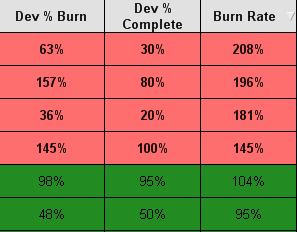# QlikView App Dev

Discussion Board for collaboration related to QlikView App Development.

Announcements
Our next Qlik Insider session will cover new key capabilities. Join us August 11th REGISTER TODAY
cancel
Showing results for
Did you mean:Not applicable

## Using a button to extract a variable's values

Hi,

I am making a straight table chart in Qlikview and I am trying to select certain values in the chart by using a button.

My chart looks like the image below. Burn rate comes from the variable 'vBurnRate' which comes from dividing Dev % Burn by Dev % Complete. It is currently \$(vBurnRate) in the expression. The colors are indicated by greater than or less than percentages. For example red is if the Burn Rate is <85% and also if it is >115%. It is yellow if it is 85-89% and also if it is 111-115%. It is green if it is 90-110%.I am trying to make a button that can select, based on Burn Rate, if it is red, yellow, or green based on the percentage range above.

10 RepliesMVPNot applicable
Author

The dimension I am using is the Dev % Complete which is just a number as a percent. For example 30%.

My expressions are:

- Dev % Burn:

\$(vDevBurn)

This variable's value is: (sum({\$<[Tracker.Category]={"Development"}>} [Tracker.Time Reported Actual]) / ([Developer.Dev Estimated Efforts]))

-Burn Rate:

\$(vBurnRate)

This variable's value is: \$(vDevBurn)/[Developer.Dev % Complete]

The background color expression for the fields are:

if(\$(vBurnRate)<'85%',rgb(255,110,110),

if(\$(vBurnRate)>='85%' and \$(vBurnRate)<'90%',rgb(255,255,0),

if(\$(vBurnRate)>='90%' and \$(vBurnRate)<='100%',rgb(34,139,34),

if(\$(vBurnRate)>='101%' and \$(vBurnRate)<='110%',rgb(34,139,34),

if(\$(vBurnRate)>='111%' and \$(vBurnRate)<='115%',rgb(255,255,0),

If(\$(vBurnRate)>'115%',rgb(255,110,110)

))))))MVP

Can you try this in a list box expression:

Aggr(If(\$(vBurnRate) < '85%', 'Red',

If(\$(vBurnRate)>='85%' and \$(vBurnRate)<'90%', 'Yellow',

If(\$(vBurnRate)>='90%' and \$(vBurnRate)<='100%', 'Green',

If(\$(vBurnRate)>='101%' and \$(vBurnRate)<='110%', 'Green',

If(\$(vBurnRate)>='111%' and \$(vBurnRate)<='115%', 'Yellow',

If(\$(vBurnRate)>'115%', 'Red')))))), [Dev % Complete])MVP

Hi,

Can you check the variable(vBurnRate) value shows with % or only numbers ? like 85% or 0.85?

may be try this..

if(\$(vBurnRate)<0.85,rgb(255,110,110),
if(\$(vBurnRate)>=0.85 and \$(vBurnRate)<0.9,rgb(255,255,0),
if(\$(vBurnRate)>=0.9 and \$(vBurnRate)<=1,rgb(34,139,34),
if(\$(vBurnRate)>=1.01 and \$(vBurnRate)<=1.1,rgb(34,139,34),
if(\$(vBurnRate)>=1.11 and \$(vBurnRate)<=1.15,rgb(255,255,0),
If(\$(vBurnRate)>1.15,rgb(255,110,110)
))))))Not applicable
Author

I cannot use a list box because I have two other fields that are in my chart. I have names of developers and a WR # they are working on. When I did try it, only a few numbers connected to the colors.Not applicable
Author

It works with both % and with numbers. When I tried <85% it worked and when I tried <0.85 it worked as well.Partner

Hi,

You can try this logic,

PFA.MVP & LuminaryNot applicable
Author

That did not work.Community Browser